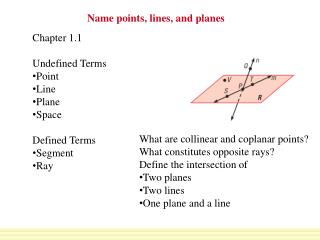DownloadDownload PresentationName points, lines, and planes

# Name points, lines, and planes

Download Presentation## Name points, lines, and planes

- - - - - - - - - - - - - - - - - - - - - - - - - - - E N D - - - - - - - - - - - - - - - - - - - - - - - - - - -
##### Presentation Transcript

1. Name points, lines, and planes • Chapter 1.1 • Undefined Terms • Point • Line • Plane • Space • Defined Terms • Segment • Ray • What are collinear and coplanar points? • What constitutes opposite rays? • Define the intersection of • Two planes • Two lines • One plane and a line

2. a. Give two other names for PQand for plane R. • Other names for PQare QPand line n. Other names for plane Rare plane SVT and plane PTV. EXAMPLE 1 Name points, lines, and planes SOLUTION

3. b. Name three points that are collinear. Name four points that are coplanar. EXAMPLE 1 Name points, lines, and planes b. Points S, P, and Tlie on the same line, so they are collinear. Points S, P, T,andVlie in the same plane, so they are coplanar.

4. 1. Use the diagram in Example 1. Give two other names for ST. Name a point that is not coplanar with points Q, S, and T. ANSWER Other names for ST are TS and PT. Point V is not coplanar with points Q,S and T for Example 1 GUIDED PRACTICE

5. a. Give another name for GH . a. Another name for GHis HG . b. The rays with endpoint Jare JE, JG, JF, and JH . The pairs of opposite rays with endpoint Jare JEand JF, and JGand JH. EXAMPLE 2 Name segments, rays, and opposite rays b. Name all rays with endpoint J . Which of these rays are opposite rays? SOLUTION

6. 2. Give another name for EF ANSWER Another name for EF is FE 3. Are HJand JH the same ray ? Are HJand HGthe same ray? Explain. ANSWER No; HJand JH have different endpoints, so they are not the same ray Yes; points J and G lie on the same side of H, so they are the same ray for Example 2 GUIDED PRACTICE

7. c. a. b. EXAMPLE 3 Sketch intersections of lines and planes a. Sketch a plane and a line that is in the plane. b. Sketch a plane and a line that does not intersect the plane. c. Sketch a plane and a line that intersects the plane at a point. SOLUTION

8. STEP 1 Draw: a vertical plane. Shade the plane. STEP 2 Draw: a second plane that is horizontal. Shade this plane a different color. Use dashed lines to show where one plane is hidden. STEP 3 Draw: the line of intersection. EXAMPLE 4 Sketch intersections of planes Sketch two planes that intersect in a line. SOLUTION

9. ANSWER Use the diagram at the right. 5. Name the intersection of PQand line k. ANSWER Point M for Examples 3 and 4 GUIDED PRACTICE • Sketch two different lines that intersect a plane at the same point.

10. ANSWER LineK Use the diagram at the right. ANSWER LineK for Examples 3 and 4 GUIDED PRACTICE 6. Name the intersection of plane A and plane B. 7. Name the intersection of line kand plane A.